# Chart

## Overview

### Chart format

Labii support the chart of the following types:
• Bar chart - presents categorical data with rectangular bars with heights or lengths proportional to the values that they represent.
• Stacked bar chart - The stacked bar chart (aka stacked bar graph) extends the standard bar chart from looking at numeric values across one categorical variable to two.
• Line chart - displays information as a series of data points called 'markers' connected by straight line segments.
• Monotone line chart - displays smooth curves between points
• Area chart - displays graphically quantitative data.
• Stacked area chart - Stacked Area Chart is plotted in the form of several area series stacked on top of one another.
• Percent area chart - A percent stacked area chart is used to depict the series of data points with positive and negative values shown overtime to reveal values of multiple series for the same data point.
• Pie chart - a circular statistical graphic, which is divided into slices to illustrate numerical proportion.
• Scatter chart - A scatter plot (aka scatter chart, scatter graph) uses dots to represent values for two different numeric variables.
• Joint line scatter - Connect each dots with straight line segments.
• Scatter with fit - Predicts the results with Least Squares Regression.

### Configuration

For each of the widget, the following parameters can be configured:
• Table - Select a table to update the filter dropdown.
• Filter - You can specify a filter to limit the results. The default setting will use the complete data set if the filter is not specified.
• Height - Chart height in px. This height is different to the widget height.

### Widgets

Here are a list of widgets under the chart category:
• Record Summary By Date
• Record Summary By Tables
• Record Summary By Projects
• Record Summary By Users
• Chart By Category X
• Chart By Numeric X

## Record Summary By Date

The widget allows you to visualize a summary of records grouped by date.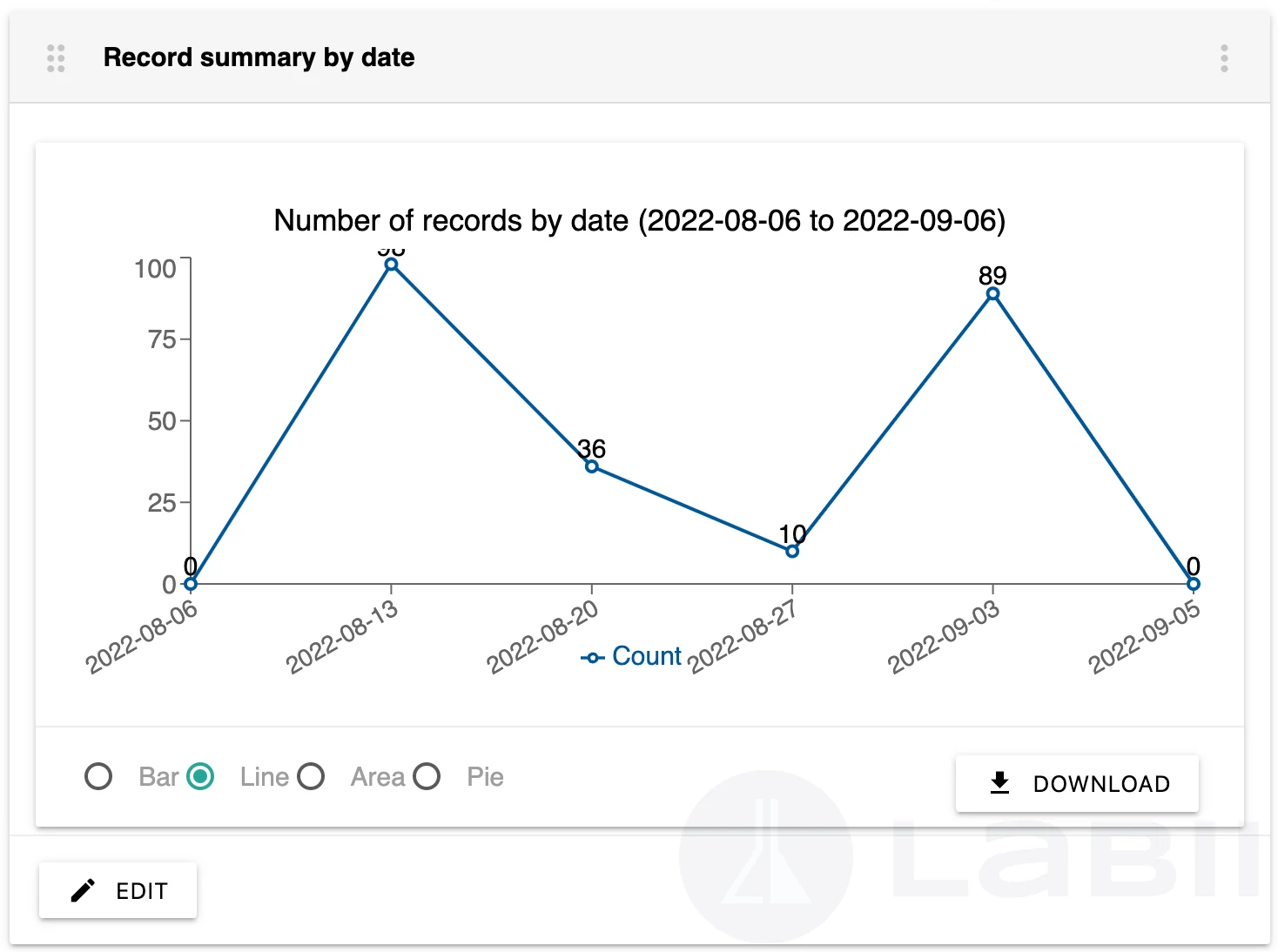Configuration:
• Time unit - Time unit for summary. Choose of Days, Weeks, Months, Years
• Time points - The number of time points that should be displayed.
• Value type - Type of value to display.
• New records
• Total records

## Record Summary By Tables

The widget allows you to visualize a summary of records grouped by tables.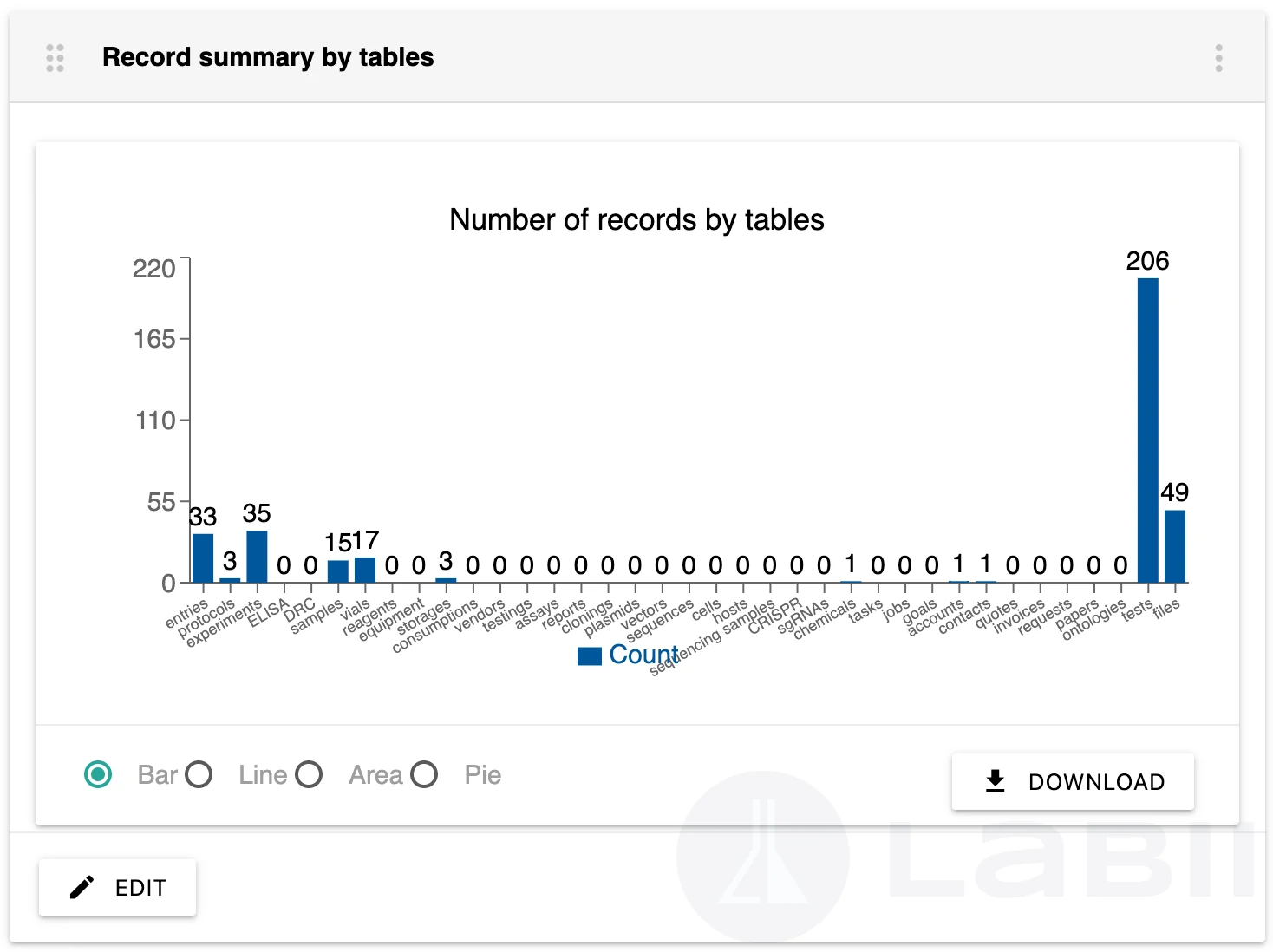## Record Summary By Projects

The widget allows you to visualize a summary of records grouped by projects.
Configuration:
• Projects. Choose a list of projects. All projects will be included if not selected.
• Tables. Choose a list of tables. All tables will be included if not selected.
• Query. Further queries to narrow the results. To exclude archived records, use is_archived=false.
• Height. Chart height in px. Default to 250px.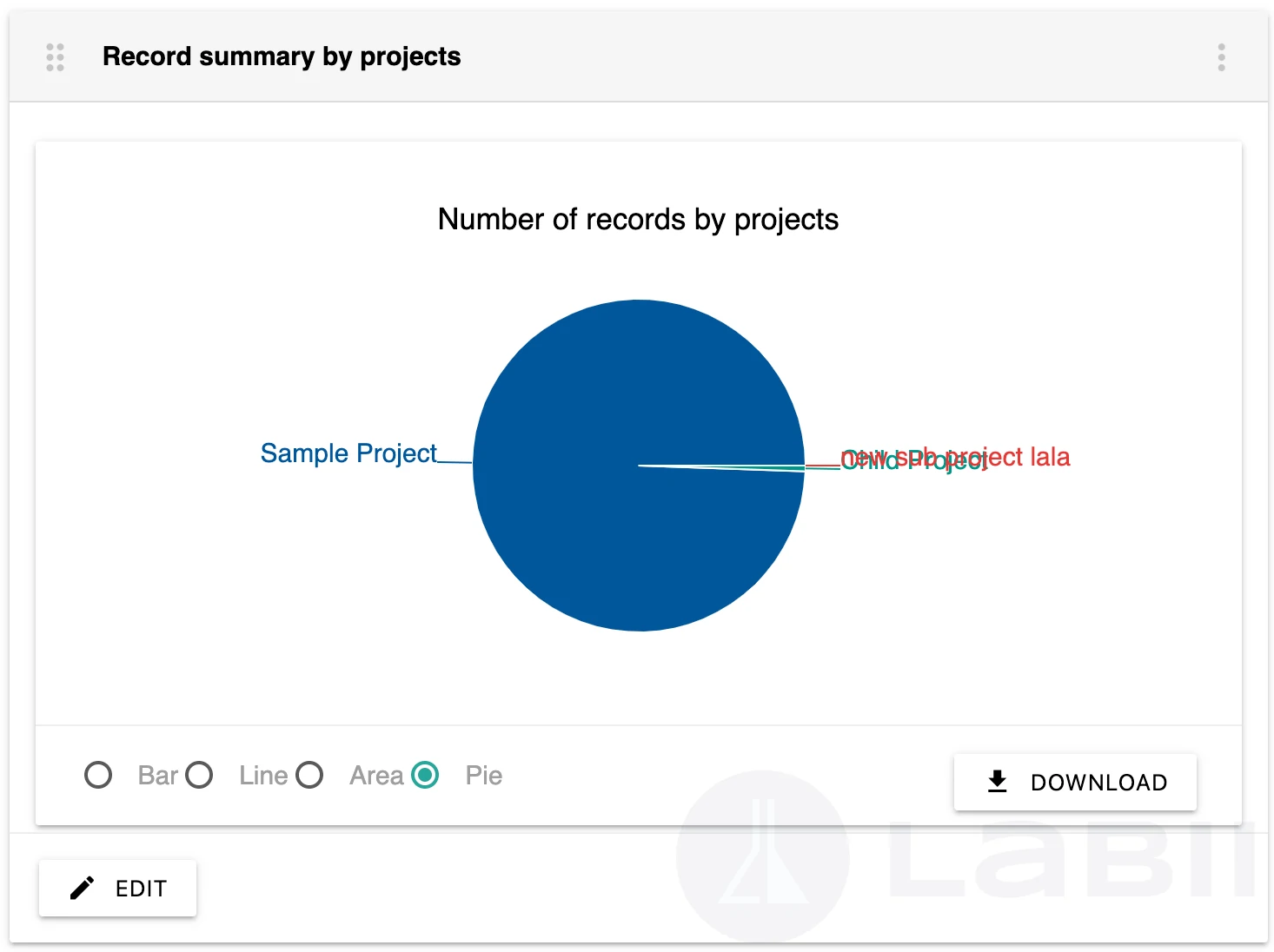## Record Summary By Users

The widget allows you to visualize a summary of records grouped by users.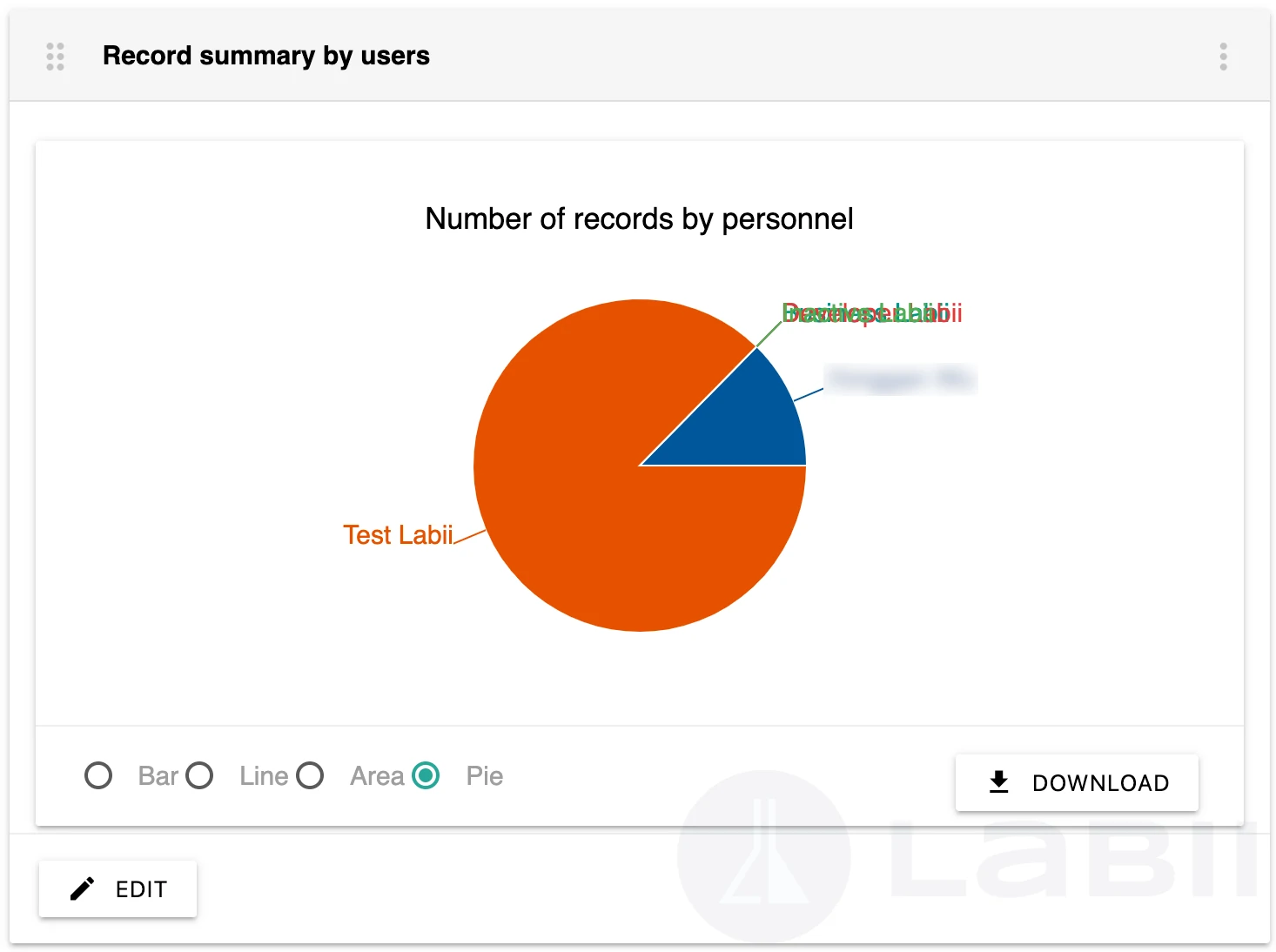## Chart By Category X

You can generate charts with category x values based on a different number of columns by using this widget.
Requirements:
• One column with Select or ForeignKey widget.
• One or more columns with Number or Formula widget. There should be a numeric value returned by the formula widget.
Configuration:
1. 1.
Select a column for the X axis. Number or Formula widgets must be used in the column.
2. 2.
Choose one or more columns for the data series. Number or Formula widgets must be used in the column.
3. 3.
Select data aggregation method. Data aggregation is the process where raw data is gathered and expressed in a summary form.
1. 1.
Sum
2. 2.
Average
3. 3.
Max
4. 4.
Median
5. 5.
Min
4. 4.
Provide the title of the chart.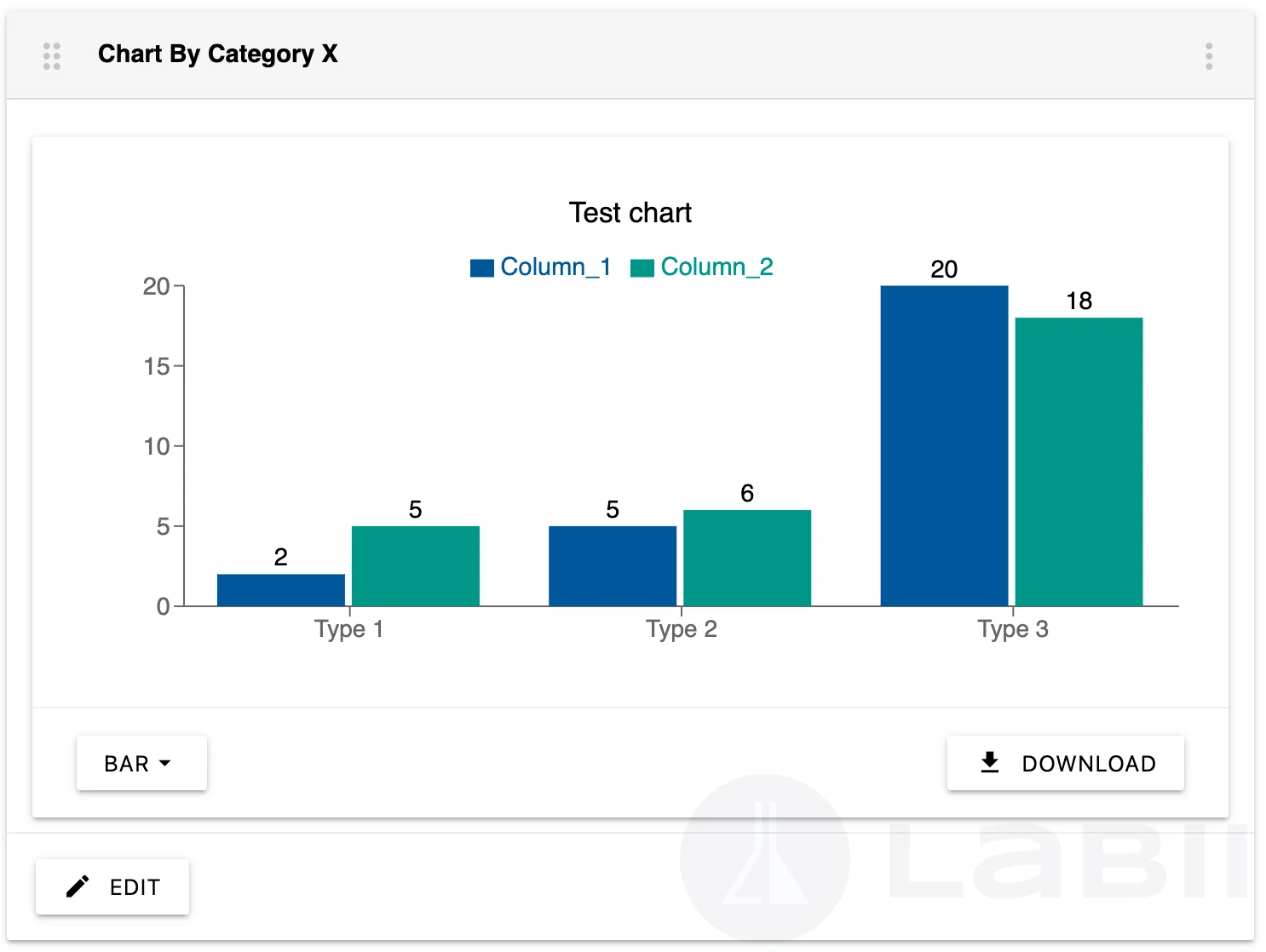## Chart By Numeric X

You can generate scatter charts with numeric x values based on a different number of columns by using this widget.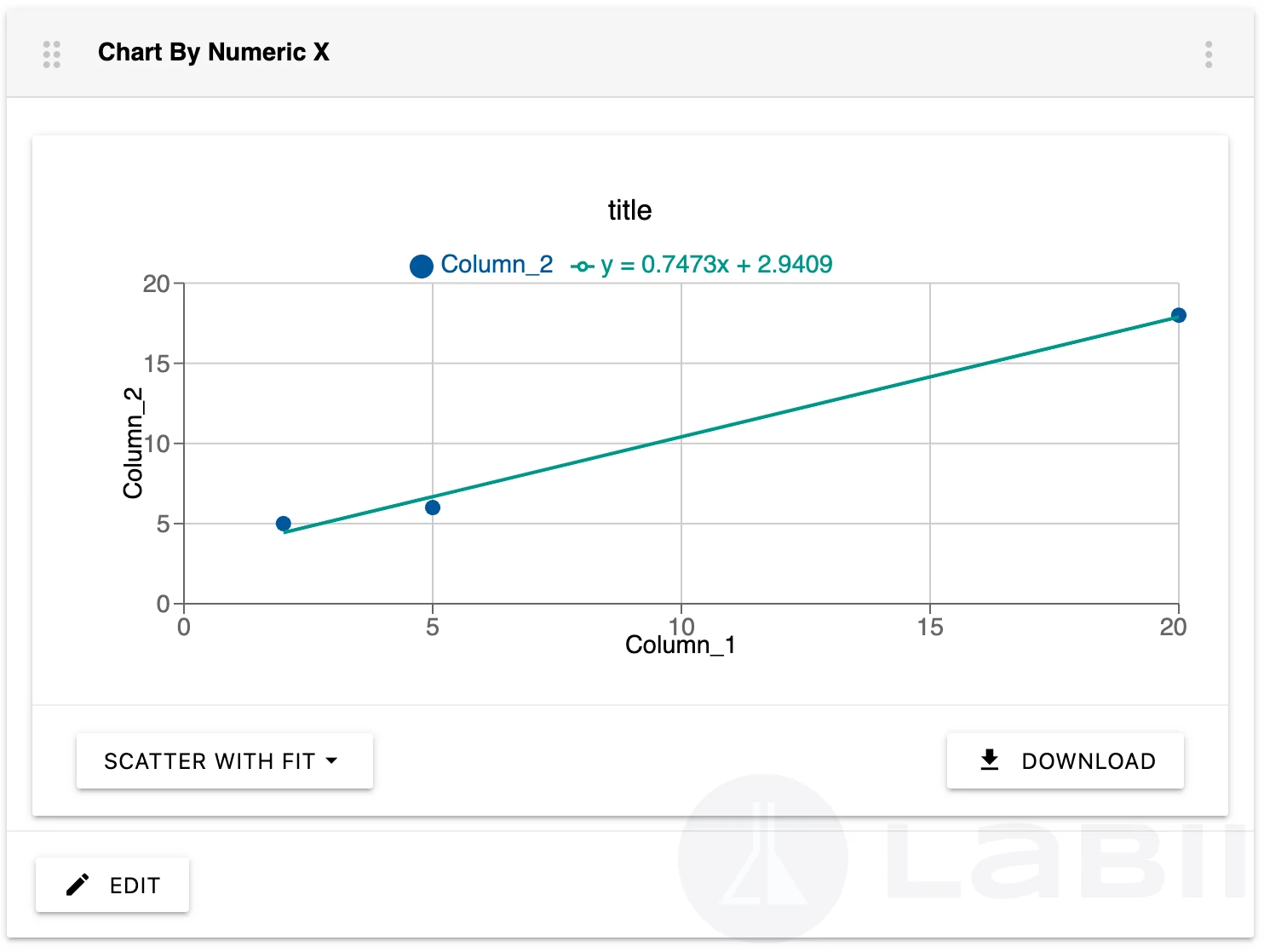Requirements:
• One column with Number or Formula widget as X axis. There should be a numeric value returned by the formula widget.
• One column with Number or Formula widget as Y axis. There should be a numeric value returned by the formula widget.
Configuration:
1. 1.
Select a column for the X axis. Number or Formula widgets must be used in the column.
2. 2.
Select a column for the data series. Number or Formula widgets must be used in the column.
3. 3.
Provide the title of the chart.
R-squared
In statistics, the coefficient of determination, abbreviated as R2 or R2 and pronounced "R squared", represents the proportion of variation in the dependent variable that can be predicted from the variation in the independent variable.
"Scatter with fit" is a tool that allows you to generate the best fit for the scatters. You will be able to see the best fit line as well as the formula on the screen.